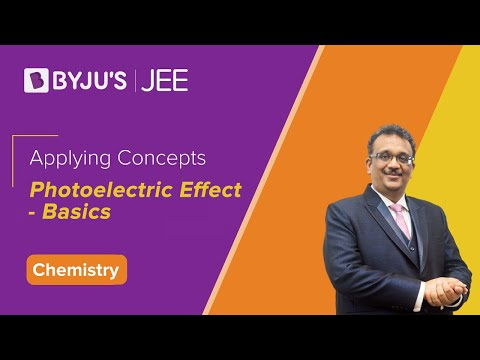# Einstein's Explanation Of Photoelectric EffectThe photoelectric effect is a phenomenon where electrons are emitted from the metal surface when light of sufficient frequency is incident upon it. The concept of the photoelectric effect was first documented in 1887 by Heinrich Hertz and later by Lenard in 1902. But both the observations of the photoelectric effect could not be explained by Maxwell’s electromagnetic wave theory of light. Hertz (who had proved the wave theory) himself did not pursue the matter as he felt sure that it could be explained by the wave theory. However, the concept failed in the following accounts:

1. According to the wave theory, energy is uniformly distributed across the wavefront and is dependent only on the intensity of the beam. This implies that the kinetic energy of electrons increases with light intensity. However, the kinetic energy was independent of light intensity.
2. Wave theory says that light of any frequency should be capable of ejecting electrons. But electron emission occurred only for frequencies larger than a threshold frequency (ν0).
3. Since energy is dependent on intensity according to wave theory, the low-intensity light should emit electrons after some time so that the electrons can acquire sufficient energy to get emitted. However, electron emission was spontaneous no matter how small the intensity of light.

Following is the table with links of other experiment related to the photoelectric effect:

## Einstein’s Explanation of Photoelectric Effect

Einstein resolved this problem using Planck’s revolutionary idea that light was a particle. The energy carried by each particle of light (called quanta or photon) is dependent on the light’s frequency (ν) as shown:

E = hν

Where h = Planck’s constant = 6.6261 × 10-34 Js.

Since light is bundled up into photons, Einstein theorized that when a photon falls on the surface of a metal, the entire photon’s energy is transferred to the electron.

A part of this energy is used to remove the electron from the metal atom’s grasp and the rest is given to the ejected electron as kinetic energy. Electrons emitted from underneath the metal surface lose some kinetic energy during the collision. But the surface electrons carry all the kinetic energy imparted by the photon and have the maximum kinetic energy.

We can write this mathematically as:

Energy of photon

= energy required to eject an electron (work function) + Maximum kinetic energy of the electron

E = W + KE

hv = W + KE

KE = hv – w

At the threshold frequency, ν0 electrons are just ejected and do not have any kinetic energy. Below this frequency, there is no electron emission. Thus, the energy of a photon with this frequency must be the work function of the metal.

w = hv0

Thus, Maximum kinetic energy equation becomes:

KE = 1/2mv2max=hv–hv0

1/2mv2max=h(v−v0)

Vmax is the maximum kinetic energy of the electron. It is calculated experimentally using the stopping potential. Please read our article on Lenard’s observations to understand this part.

Stopping potential = ev0 = 1/2mv2max

Thus, Einstein explained the Photoelectric effect by using the particle nature of light.

The below video is a quick revision of what is photoelectric effect:## Frequently Asked Questions – FAQs

Q1

### Who discovered electron?

J J Thomson discovered electron.

Q2

### What is the threshold frequency?

Threshold frequency is defined as the minimum frequency that is required for the radiation such that photoelectric effect is produced.

Q3

### Which metals exhibit photoelectric effect?

Alkali metal is usually used for exhibiting photoelectric effect. Metals such as copper and sodium are examples of metals that exhibit photoelectric effect.

Q4

### What type of light is needed to cause the photoelectric effect?

Ultraviolet light is the type of light that is needed to cause the photoelectric effect.

Q5

### What are the applications of photoelectric effect?

The applications of photoelectric effect are electric eye in door openers, light meters in photography, photostatic copying, and solar panels.

Test Your Knowledge On Einsteins Explanation!